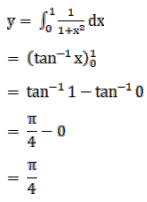# Mark against the correct answer in the following:Question:

Mark $(\sqrt{)}$ against the correct answer in the following:

$\int_{0}^{1} \frac{1}{\left(1+x^{2}\right)} d x=?$

A. $\frac{\pi}{2}$

B. $\frac{\pi}{3}$

C. $\frac{\pi}{4}$

D. none of these

Solution: